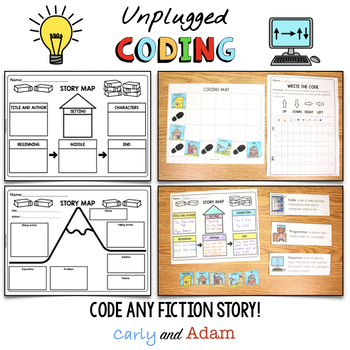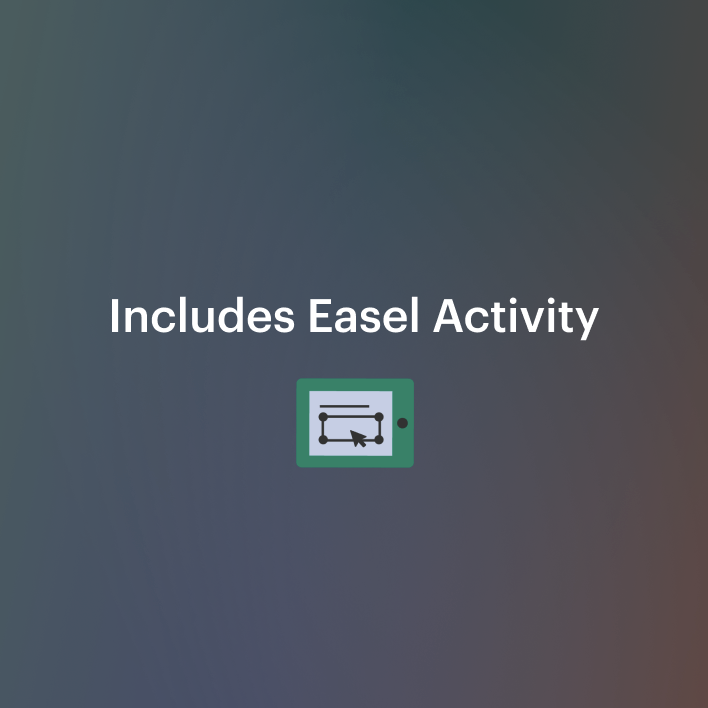DID YOU KNOW:
Seamlessly assign resources as digital activities

Learn how in 5 minutes with a tutorial resource. Try it Now

Learn More# Code a Story Unplugged Coding Activity TpT Digital Distance LearningK - 3rd, Homeschool
Standards
Resource Type
Formats Included
• PDF
•Activity
Pages
29 pages
Easel Activity Included
This resource includes a ready-to-use interactive activity students can complete on any device. Easel by TpT is free to use! Learn more.

### Description

Includes both PDF and TpT Digital Versions for Google Classroom Distance Learning and in-person STEM classes.

Retell a story using coding!

In this unplugged coding activity, Students create a story map of any fictional story. They use their story map to code the sequence of events that happen in the story.

New to Coding?

No worries! Coding can be intimidating, but you don't have to have a background in computer science to teach coding. This resource is designed to be so simple a sub could use it. Get started with coding today! Perfect activity for Hour of Code.

Coding Process:

Students learn the logic and thinking that goes into writing codes using Directional Coding. They focus on 3 vocabulary words: Programmer, Code, and Sequence.

Students connect coding and sequencing to retelling stories. After students write their code, they use it to retell the story with their partner. At the end, students reflect on what they learned using the included reflection pages.

Material List:

• Scissors
• Paper
• Any Fiction Story (Example, 3 Little Pigs)

Includes:

• Teacher Instructions (with differentiation and photos)
• Student Instructions
• Story Map (2 Difficulty Options)
• Student Coding Journal
• Student Unplugged Coding Activity (Includes Partner Coding Practice)
• Student Reflection Sheet
• Coding Vocabulary Words
• CSTA and Common Core Standards
• TpT Digital Version for Distance Learning with Google Classroom

Digital Activity:

To use Easel for Distance Learning, select "Open in Easel" on this listing.

Aligns to CSTA (Computer Science Teachers Association) and Common Core.

You may also like...

Intro to Unplugged Coding: Binary Code

Instagram

CSTA STANDARDS:

1A-AP-08 Model daily processes by creating and following algorithms

(sets of step-by-step instructions) to complete tasks.

1A-AP-09 Model the way programs store and manipulate data by using numbers or other symbols to represent information.

1A-AP-11 Decompose (break down) the steps needed to solve a problem into a precise sequence of instructions.

Total Pages
29 pages
N/A
Teaching Duration
1 hour
Report this Resource to TpT
Reported resources will be reviewed by our team. Report this resource to let us know if this resource violates TpT’s content guidelines.

### Standards

to see state-specific standards (only available in the US).
Look for and express regularity in repeated reasoning. Mathematically proficient students notice if calculations are repeated, and look both for general methods and for shortcuts. Upper elementary students might notice when dividing 25 by 11 that they are repeating the same calculations over and over again, and conclude they have a repeating decimal. By paying attention to the calculation of slope as they repeatedly check whether points are on the line through (1, 2) with slope 3, middle school students might abstract the equation (𝑦 – 2)/(𝑥 – 1) = 3. Noticing the regularity in the way terms cancel when expanding (𝑥 – 1)(𝑥 + 1), (𝑥 – 1)(𝑥² + 𝑥 + 1), and (𝑥 – 1)(𝑥³ + 𝑥² + 𝑥 + 1) might lead them to the general formula for the sum of a geometric series. As they work to solve a problem, mathematically proficient students maintain oversight of the process, while attending to the details. They continually evaluate the reasonableness of their intermediate results.
Look for and make use of structure. Mathematically proficient students look closely to discern a pattern or structure. Young students, for example, might notice that three and seven more is the same amount as seven and three more, or they may sort a collection of shapes according to how many sides the shapes have. Later, students will see 7 × 8 equals the well remembered 7 × 5 + 7 × 3, in preparation for learning about the distributive property. In the expression 𝑥² + 9𝑥 + 14, older students can see the 14 as 2 × 7 and the 9 as 2 + 7. They recognize the significance of an existing line in a geometric figure and can use the strategy of drawing an auxiliary line for solving problems. They also can step back for an overview and shift perspective. They can see complicated things, such as some algebraic expressions, as single objects or as being composed of several objects. For example, they can see 5 – 3(𝑥 – 𝑦)² as 5 minus a positive number times a square and use that to realize that its value cannot be more than 5 for any real numbers 𝑥 and 𝑦.
Attend to precision. Mathematically proficient students try to communicate precisely to others. They try to use clear definitions in discussion with others and in their own reasoning. They state the meaning of the symbols they choose, including using the equal sign consistently and appropriately. They are careful about specifying units of measure, and labeling axes to clarify the correspondence with quantities in a problem. They calculate accurately and efficiently, express numerical answers with a degree of precision appropriate for the problem context. In the elementary grades, students give carefully formulated explanations to each other. By the time they reach high school they have learned to examine claims and make explicit use of definitions.
Use appropriate tools strategically. Mathematically proficient students consider the available tools when solving a mathematical problem. These tools might include pencil and paper, concrete models, a ruler, a protractor, a calculator, a spreadsheet, a computer algebra system, a statistical package, or dynamic geometry software. Proficient students are sufficiently familiar with tools appropriate for their grade or course to make sound decisions about when each of these tools might be helpful, recognizing both the insight to be gained and their limitations. For example, mathematically proficient high school students analyze graphs of functions and solutions generated using a graphing calculator. They detect possible errors by strategically using estimation and other mathematical knowledge. When making mathematical models, they know that technology can enable them to visualize the results of varying assumptions, explore consequences, and compare predictions with data. Mathematically proficient students at various grade levels are able to identify relevant external mathematical resources, such as digital content located on a website, and use them to pose or solve problems. They are able to use technological tools to explore and deepen their understanding of concepts.
Model with mathematics. Mathematically proficient students can apply the mathematics they know to solve problems arising in everyday life, society, and the workplace. In early grades, this might be as simple as writing an addition equation to describe a situation. In middle grades, a student might apply proportional reasoning to plan a school event or analyze a problem in the community. By high school, a student might use geometry to solve a design problem or use a function to describe how one quantity of interest depends on another. Mathematically proficient students who can apply what they know are comfortable making assumptions and approximations to simplify a complicated situation, realizing that these may need revision later. They are able to identify important quantities in a practical situation and map their relationships using such tools as diagrams, two-way tables, graphs, flowcharts and formulas. They can analyze those relationships mathematically to draw conclusions. They routinely interpret their mathematical results in the context of the situation and reflect on whether the results make sense, possibly improving the model if it has not served its purpose.